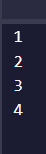# Fibonacci challenge

please what is wrong with this code below. i keep getting a number less than the answer

see below link to the challenge

``````function sumFibs(num) {
let prev = 0;
let result = 0;
for (let i = 1; i <= num; i=i+prev){
if (i % 2 == 1){
result+=i
}
prev = i - prev;
}
return result;
}

console.log(sumFibs(4));
``````
``````  **Your browser information:**
``````

User Agent is: `Mozilla/5.0 (Macintosh; Intel Mac OS X 10_15_7) AppleWebKit/537.36 (KHTML, like Gecko) Chrome/100.0.4896.75 Safari/537.36`

Challenge: Sum All Odd Fibonacci Numbers

I am not sure that is fibonacci sequence:it should be 1,1,2,3,5
(I have added `console.log(i)` inside your loop)

please what could i have done differently?

you need to calculate the fibonacci series correctly

i have really struggled to understand how i am going to calculate this series correctly. i have looked at links, watched youtube videos on this and i haven’t been able to.

i just cannot wrap my head around this one

Don’t worry about fancy code for fibbonaci numbers you may have seen elsewhere.

Let’s look at the definition given:

Every additional number in the sequence is the sum of the two previous numbers.

So, can you finish this:

``````let fib1 = 1;
let fib2 = 1;
let fib3; // how do you set this value with fib1 and fib2?
``````
``````let fib2 = 1;
let fib3 = fib1 + fib2; // how do you set this value with fib1 and fib2?
``````

could this fib3 be the sum?

Yup. Now how can this be wrapped into a loop to compute the fibbonaci numbers less than 50? Something like this?

``````let fib1 = 1;
let fib2 = 1;
let fib3 = fib1 + fib2;

while (....) {

}
``````

Once you add in a loop, you are actually most of the way to a solution for this problem.

let fib1 = 1;
let fib2 = 1;
let fib3 = fib1 + fib2;

while (fib2 <=50) {

}

``````i love using the for loop and that is what initially came to mind. i did try
``````

for (let i = 1; i <=50; i++)

``can it work in place of the while loop?``

A `for` loop is for when you run the loop body a fixed number of times? A `while` loop is for when you need to iterate until a condition is met. Which is closer to the challenge requirements?

well understood. After the while loop then?

Well, something needs to happen in that loop. How can you use the loop to make more fibbonaci numbers?

i need to keep on adding fib1 to fib2 to keep getting fib3. it’s how to increment fib1 and fib2 that is the issue now

You want to repeat this line right?

But you need to update the values in fib1 and fib2. After you have competed fib3, which two numbers do you need to add together?

fib1 = fib2
fib2 = fib3 - fib2

my thoughts

Why this part

i am trying to increment fib2. i would have written fib2 = fib3 - fib1, but since fib1 is now equal to fib2 i used the fib2

But why. Why not do what you did to increment fib1?

1 Like

let fib1 = 1
let fib2 = 0
let fib3 = fib1 + fib2

ooh like
fib2 = fib3

this works